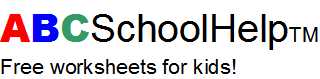Back | Print page | Home

# Grade 1 Math: Numbers 5

```Write the answers:

a. One more than eight

b. One more than sixty-two

c. One more than sixty-nine

d. Ten more than four

e. Ten more than fifty-eight

f. One less than twelve

g. One less than eighty

h. Ten less than twelve

i. Ten less than eighty

j. Ten less than forty-three
```